# Thales' Theorem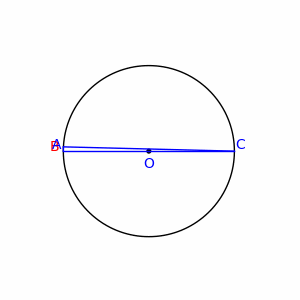Image Credits: Wikipedia

Thales' Theorem is a simple yet important geometrical result which describes the relationship between inscribed right angles and circles. A special case of the inscribed angle theorem, Thales' Theorem is as follows:

Let $A$ and $C$ be two points on the circumference of some circle $S$ such that $\overline{AC}$ is a diameter of $S$. If a point $B$ is placed anywhere on the circumference of $S$ (other than $A$ or $C$), then $\angle ABC$ will always be a right angle.

Using the inscribed angle theorem, it also follows that the reverse of this statement holds. Given any 3 points $A,B,C$ on the circumference of $S$ such that $\angle ABC=90^{\circ}$, $\overline{AC}$ must be a diameter of the circle. We now present two different approaches to proving Thales'.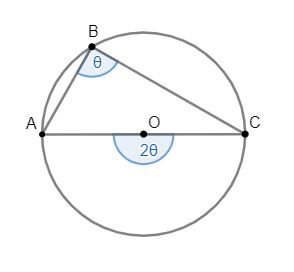A proof via inscribed angle theorem.

Consider a circle $S$ with center $O$ and two diametrically opposite points $A,C$ on the circumference of$S$. Since points $A,C,O$ are all co-linear, it follows that $\angle AOC=180^{\circ}$.

By the inscribed angle theorem, we know that any angle $\theta$ inscribed in $S$ will be half of the central angle which subtends the same arc on $S$. In other words, if a point $B$ is placed on the circumference of $S$, then angle $\angle ABC$ will be half of angle $\angle AOC$. Since we have that $\angle AOC=180^{\circ}$, it follows that $\angle ABC=90^{\circ}$

Thales' theorem has many elegant applications to pure geometry. However, since Thales' often arises in coordinate geometry, let's take a look at a proof of Thales' which uses just that.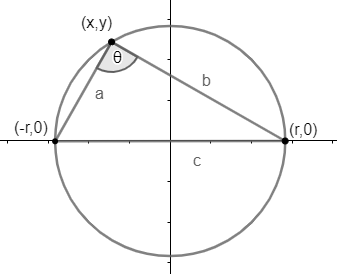A proof via coordinate geometry.

Consider a circle $S$ of radius $r$ located at the origin. Notice that $S$ is described by the equation $x^2+y^2=r^2$. Consider the two diametrically opposite points $(-r,0)$ and $(r,0)$ located at the intersection of $S$ with the $x$-axis. Place a third point anywhere on the upper half of the circumference of $S$.

Pythagoras' theorem guarantees that in a triangle with side lengths $a,b,c$, with $c$ being the longest side, $a^2+b^2=c^2$ if and only if the triangle is a right triangle. Therefore, we will show that $\theta=90^{\circ}$ in the diagram to the right by showing that $a^2+b^2=c^2$.

Using Pythagoras' theorem, we can determine the legnths of each side as follows

\begin{aligned} a^2&=(x-(-r))^2+(y-0)^2=x^2+2xr+r^2+y^2\\b^2&=(x-r)^2+(y-0)^2=x^2-2xr+r^2+y^2\\c^2&=(r-(-r))^2=4r^2\end{aligned}

Next, observe that

$a^2+b^2=(x^2+2xr+r^2+y^2)+(x^2-2xr+r^2+y^2)=2x^2+2y^2+2r^2=2(x^2+y^2)+2r^2$

Recall that since the point $(x,y)$ was chosen on the circumference of $S$, it must satisfy $x^2+y^2=r^2$. Using this to simplify the equation above:

$a^2+b^2=2(x^2+y^2)+2r^2=2(r^2)+2r^2=4r^2=c^2$

Since we have shown that $a^2+b^2=c^2$, we have also proved that $\theta=90^{\circ}$ which finishes our proof of Thales'.

There are many other ways to prove Thales', some using pure geometry, some using trigonometry, and some even using complex numbers! We encourage you to explore and try to come up with your own unique proof of Thales'. If you do manage to come up with your own proof, great! Just don't get too excited and sacrifice an ox (see entry "Thales' theorems" on this page for more details).

Here are a couple problems centered around Thales' for practice. The first few aren't too bad, but be careful, the last one is quite tricky!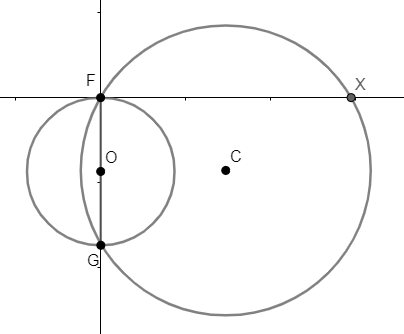Circle $S$ is placed in the 2D-plane with radius $3$ and its center $O$ placed on the $y$-axis. $S$ intersects the $y$-axis at both the point $G$ and the origin (labeled $F$).

Circle $T$ is then drawn with twice the radius of circle $S$ such that $T$ also intersects $F$ and $G$.

If circle $T$ intersects the $x$-axis at points $X$ and $G$, find the length of segment $\overline{FX}$.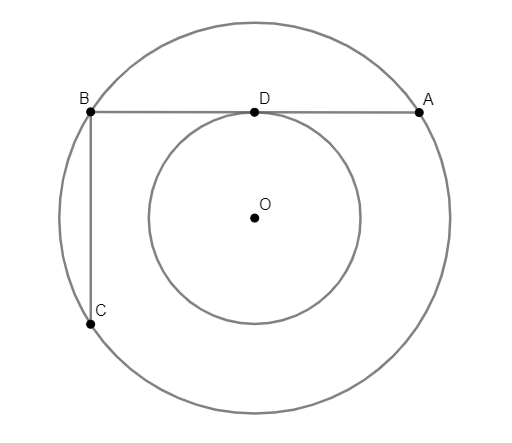Circles $S$ and $T$ are co-centric at point $O$ with circle $S$ inside circle $T$. Line segment $\overline{BA}$ is a cord of circle $T$ and is tangent to circle $S$ at point $D$. Line segment $\overline{BC}$ is a cord of circle $T$ and is perpendicular to segment $BA$.

If $\overline{BC}$ has length 1 and $\overline{BA}$ has length 2, find the radius of the inner circle $S$

This last one can be a bit tricky.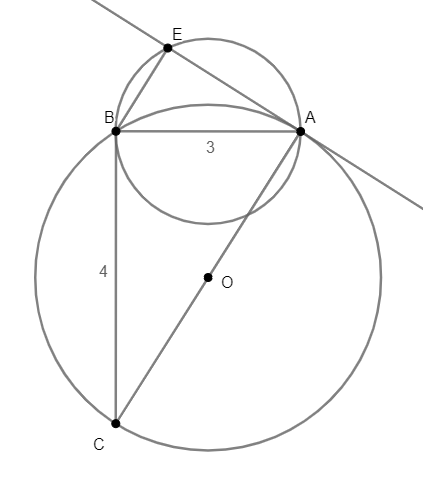Circle $T$ is drawn with perpendicular cords $\overline{AB}$ and $\overline{BC}$ with lengths 3 and 4, respectively. Circle $S$ is then drawn such that $\overline{AB}$ is a diameter of $S$. Finally, a line is drawn through point $A$ perpendicular to $\overline{AC}$. Let point $E$ be the other intersection of this line with circle $S$.

Find the length of segment $\overline{BE}$.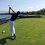Note by Trevor Arashiro
1 year, 2 months ago

This discussion board is a place to discuss our Daily Challenges and the math and science related to those challenges. Explanations are more than just a solution — they should explain the steps and thinking strategies that you used to obtain the solution. Comments should further the discussion of math and science.

When posting on Brilliant:

• Use the emojis to react to an explanation, whether you're congratulating a job well done , or just really confused .
• Ask specific questions about the challenge or the steps in somebody's explanation. Well-posed questions can add a lot to the discussion, but posting "I don't understand!" doesn't help anyone.
• Try to contribute something new to the discussion, whether it is an extension, generalization or other idea related to the challenge.

MarkdownAppears as
*italics* or _italics_ italics
**bold** or __bold__ bold
- bulleted- list
• bulleted
• list
1. numbered2. list
1. numbered
2. list
Note: you must add a full line of space before and after lists for them to show up correctly
paragraph 1paragraph 2

paragraph 1

paragraph 2

[example link](https://brilliant.org)example link
> This is a quote
This is a quote
    # I indented these lines
# 4 spaces, and now they show
# up as a code block.

print "hello world"
# I indented these lines
# 4 spaces, and now they show
# up as a code block.

print "hello world"
MathAppears as
Remember to wrap math in $$ ... $$ or $ ... $ to ensure proper formatting.
2 \times 3 $2 \times 3$
2^{34} $2^{34}$
a_{i-1} $a_{i-1}$
\frac{2}{3} $\frac{2}{3}$
\sqrt{2} $\sqrt{2}$
\sum_{i=1}^3 $\sum_{i=1}^3$
\sin \theta $\sin \theta$
\boxed{123} $\boxed{123}$

Sort by:

For the last question, there is a slicker solution involving similar triangles ($\triangle ABC \sim \triangle BEA$), and the answer immediately follows after finding the length $AC$.

- 1 year, 2 months ago

There certainly is! However, i wrote this article assuming nothing about readers’ background in geometry. Many people learn Thales’ before similar triangles so I wanted to make sure everyone could understand it.

- 1 year, 2 months ago

@Trevor Arashiro How you made these switches of 'solution' and 'hint'?

- 1 year, 2 months ago

Yes, even I want to know!

- 1 year, 2 months ago

The last question has a solution using trigonometry as well, and the answer comes out without much effort

- 1 year, 1 month ago

But I agree that your solution is much more elegant and also useful for learners of pure geometry

- 1 year, 1 month ago